# MP Board Class 11th Chemistry Important Questions Chapter 8 Redox Reactions

## MP Board Class 11th Chemistry Important Questions Chapter 8 Redox Reactions

### Redox Reactions Important Questions

Redox Reactions Objective Type Questions

Question 1.

Question 1.
Metals used in Daniel cell are:
(a) N and Cu
(b) Zn and Ag
(c) Ag and Cu
(d) Zn and Cu
(d) Zn and Cu

Question 2.
Which of the following is strongly reducing:
(a) r
(b) cr
(c) Bf
(d) r
(d) r

Question 3.
Oxidation in electrolyte occurs:
(a) At anode
(b) At cathode
(c) At both the electrodes
(d) None of these
(a) At anodeQuestion 4.
Which of the following statement is correct? Galvanic cell converts:
(a) Chemical energy to electrical energy
(b) Electrical energy to chemical energy
(c) Electrical state of metal to combined state
(d) Electrolytes to ions
(b) Electrical energy to chemical energy

Question 5.
Oxidation number of chlorine in HOCl is:
(a) – 1
(b) 0
(c) +I
(d) +2
(c) +I

Question 6.
Oxidation number of Mn in K2MnO4 is:
(a) +2
(b) +6
(c) +7
(d) 0
(b) +6

Question 7.
Oxidation number of Cr in K2Cr2O7 is:
(a) -6
(b) +6
(c) +2
(d) -2.
(b) +6

Question 8.
Oxidation number of Ni in Ni(CO)4 is:
(a) 0
(b) +2
(c) +1
(d) – 1.
(a) 0Question 9.
Unit of cell constant is:
(a) ohm-1cm-1
(b) ohm cm
(c) cm
(d) cm-1
(d) cm-1

Question 10.
Oxidation state of nitrogen is maximum in:
(a) N3H
(b) NH2OH
(c) N2H4
(d) NH3
(a) N3H

Question 11.
Oxidation state of oxygen is zero in:
(a) CO
(b) O3
(c) SO2
(d) H2O2
(b) O3

Question 12.
Oxidation state of Fe in K4[Fe(CN)6] is:
(a) +2
(b) +6
(c) +3
(d) +4
(a) +2

Question 13.
Oxidation state of S In H2S2O8 is:
(a) +2
(b) +4
(c) +6
(d) +7
(c) +6Question 14.
Oxidation state of Mn in KMnO4 is:
(a) +4
(b) +6
(c) +7
(d) +5
(c) +7

Question 15.
oxidation state of chlorine is in:
(a) HCl
(b) HClO4
(c) ICI
(d) Cl2O
(d) Cl2O

Question 16.
Oxidation state of Cl in ClO3 ion is:
(a) +4
(b) +5
(c) +3
(d) +2
(b) +5

Question 17.
Halogen which is reduced most easily is:
(a) I2
(b) Br2
(c) Cl2
(d) F2
(a) I2

Question 18.
Oxidation state of C in CCl4 is:
(a) +4
(b) -4
(c) +6
(d) – 6
(a) +4

Question 19.
Oxidation state of S in S04-2 is:
(a) +6
(b) -6
(c) +5
(d) – 5
(a) +6Question 20.
Oxidizing agent are:
(a) Electron acceptor
(b) Electron donor
(c) Proton acceptor
(d) Neutron acceptor
(a) Electron acceptor

Question 2.
Fill in the blanks:

1. Process of loss of electrons is called ……………………..
2. Process of gain of electrons is called …………………….
3. The deterioration of metals in presence of atmospheric gases and moisture is called …………………………
4. A device in which electric energy gets converted to chemical energy is called …………………….. cell
5. In electrochemical series the ability to reduce ………………………… on moving down
6. Most strong reducing element is ………………………….
7. Most strong oxidizing element is ………………………..
8. Theory of dissociation of electrolyte was proposed by ……………………….

1. Oxidation
2. Reduction
3. Corrosion
4. Electrochemical cell
5. Decreases
6. Lithium
7. Fluorine
8. ArrheniusQuestion 3.

1. What is balanced redox reaction?
2. Why does iron displaced copper from its salt solution?
3. What is the oxidation state of chlorine in Cl2O?
4. Why does an electrolyte dissociate into ions when dissolved in water?
5. A saturated solution of KNO3 is used for the formation of salt bridge. Why?
6. In SnCl2 + 2 FeCl3 → SnCl4 + 2 FeCl2, reaction which element is oxidizing agent?
7. What is oxidation state of Xe in XeO3?

1. Redox reaction in which amount of oxidation and reduction is to the same extent.
2. Because standard eletrode potential value of iron is less then the standard electrode potential of copper.
3. +1
4. Because the electrostate attractive force between the ions break due to water.
5. Because the speed of K+ and NO3 and is almost same.
6. FeCl3
7. +6

Question 4.
Match the following: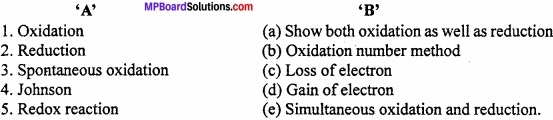1. (c)
2. (d)
3. (a)
4. (b)
5. (e)

Redox Reactions Very Short Answer Type Questions

Question 1.
Why iron displaces copper from its salt solution?
Because the standard electrode potential of iron is less than copper.

Question 2.
What is the oxidation number of Cl in Cl2O?
+1.

Question 3.
When an electrolyte is dissolved in water, it dissociates into ions? Why?
Because due to water the electrostatic attraction force between the ions breaks.

Question 4.
Why the saturated solution of KNO3 is used to prepare salt bridge?
Because the speed of K+ and NO3 ions is similar.Question 5.
In SnCl2 + 2FeCl3 → SnCl4 + 2FeCl2, which is oxidizing agent?
FeCl3.

Question 6.
What is the oxidation number of Xe in XeO3?
+6.

Question 7.
Which metal is used in Daniel cell?
Lithium.

Question 8.
What is the oxidation state of Mn in K2MnO4?
+6.

Question 9.
Which is the most reducing element?
Lithium.

Question 10.
Who maintains the neutrality among the two half cells?
Salt bridge.Question 11.
What is the standard electrode potential of SHE?
0.00V (Zero).

Question 12.
Which is the best conducting metal?
Silver (Ag).

Question 13.
The reaction 3ClOaq → Cl3 + 2Cl is an example of?
Disproportionation reaction.

Question 14.
Which halogen reduces very easily?
F2.Question 15.
Which reaction is called electron acceptance reaction?
Reduction.

Question 16.
Where oxidation occurs in electrolysis?
At anode.

Redox Reactions Short Answer Type Questions – I

Counting atoms calculator in my website step-by-step explanation.

Question 1.
What is oxidation process? Explain redox reaction with example?
Oxidation state of an element is defined as “The residual charge left on its atom”. When all the other atoms are removed from the molecule as ion.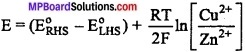2Mg + O2 → 2MgO [Addition of oxygen]
2FeCl2 → 2FeCl3 [Addition of electronegativity element]
H2S + Cl2 → S + 2HCl [Removal of hydrogen]
2Ki + H2O2 → 2KOH + I2 [Removal of electropositive element]

Question 2.
What is reduction process? Explain with example?
The ability of any substance to accept hydrogen atom or +ve electrolytic element or to release oxygen or – ve element is called reduction process.
CuO + H2 → Cu + H2O [Remove of oxygen]
2FeCl3 + H2 S → 2FeCl2 + 2HCl + S [Removal of electromagnetive element]
Cl2 + H2S → 2 HCl + S [Addition of H]
S + Fe → Fes [Addition of electropositive element]

Question 3.
AgF2 is unstable compound, but if it is formed then it will acts as strong oxidising agent Why?
In AgF2, Ag has oxidation state +2, it is highly unstable, it very easily accept electron and forms oxidation state +1. Because Ag+ has stable configuration so it is stable.
Ag2+ + e → Ag+
This is the reason why AgF2 behaves as a strong oxidizing agent.Question 4.
By the structure of S4O62-clarify that the oxidation state of S is +5?
The oxidation state of two central sulphur atom is 0. As the electron pair remains in the centre cf the bond. So the remaining sulphur atom has oxidation state 4 – 5.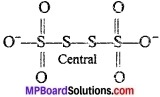⇒ 6(-2) + 2x = -2
⇒ -12 + 2x = -2
⇒ 2x = -2 + 12 = 10
⇒ x = $$\frac{10}{2}$$ = 5.

Question 5.
What is electrochemical series?
Electrochemical series:
When different elements and ions are arranged in increasing order of their standard electrode potential, a series is obtained which is called electrochemical series as activity series.

Question 6.
What is salt bridge? Write its two uses?
1. It connects the solution in two half ceil and completes the cell circuit

2. The salt bridge which contains the solution of an electrolyte say K+NO3 maintains the electrical neutrality by diffusion of ion through it. As positive ion begins to be formed in oxidation half cell negative ion from the salt bridge diffuse out into this half cell. Similarly, as the positive Ion begin to be consumed in the reduction.

Hair cell positive:
On from the salt bridge diffuse out into this half cell. As a result the solution in the two half cell remain electrically neutral and the current continous to flow.

Question 7.
On the basis of electron transfer explain oxidation and reduction reactions?
Reactions involving exchange of electrons are called redox reaction. Reaction given below
2FeCl3 + SnCl2 → 2FeCl2 + SnCl4
It is an example of redox reaction in which FeCl3 is oxidizing agent while SnCl2 is a reducing agent.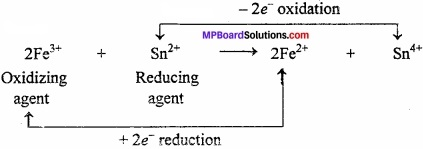According to electronic concept during oxidation substance loses one or more electron and its positive oxidation number increases while negative oxidation number decreases. (MPBoardSolutions.com) On the other hand, during reduction substance gains one or more electron and is positive oxidation number decreases while negative oxidation number increases.

Question 8.
What is electrochemical equivalent?
The mass of any substance deposited at any electrode is directly proportional to the quantity of electricity passed.

Thus, if W gm substance is deposited on passing Q coulomb electricity, then W ∝ Q or W = ZQ
Where, Z is a constant of proportionality and is called electrochemical equivalent of the substance deposited. If a current of I ampere is passed for t second, then Q = I × t. (MPBoardSolutions.com) So that, W = Z × Q = Z × I × r

Thus, if, Q = 1 coulomb, 1=1 ampere and t = 1 second, then W = Z. Hence, electrochemical equivalent of a substance may be defined as, “The mass of the substance deposited when a current of one ampere is passed for one second”.

As one Faraday (96500 C) deposits one gram equivalent of the substance, hence, electrochemical equivalent can be calculated from the equivalent mass.
$$\frac { Equivalentmassofthesubstance\quad }{ 96500 }$$Question 9.
What happens when Zn is kept in CuS04 solution? Explain with equation?
The reaction occurring in the beaker may be written asCancelling the common S042+ ions
Zn(s) + Cu2+(eq) → Zn2+(eq) + Cu(s)
In this case Zinc metal loses electron and gets oxidised to Zn++ ions which goes into the solution. Due to this, weight of zinc plate gradually decreases. On the other hand, electrons lost by Zinc atom are taken up by Cu2+ ions of CuS04 solution.

Question 10.
What do you mean by standard electrode potential?
Standard electrode potential: Standard electrode potential (E°) of a half cell (electrode) is the potential difference which is produced when one electrode is dipped in the solution of molar concentration of its ion at 298 K. (MPBoardSolutions.com) If electrode is gaseous, the pressure of gas must be one atmosphere.
In IUPAC system reduction potentials are known as standard electrode potential.

Question 11.
The reaction Cl2(g) + 2OH(aq) → ClO(aq) + Cl(aq) + H2O(l) represents the process of bleaching. Identify and name species that bleaches the substances due to its oxidizing action?In this reaction, O. N. of Cl increases from 0 in Cl2 to +1 in ClO and decreases to -1 in Cl. Therefore, Cl2 is both oxidized to ClO and reduced to Cl (Disproportionation reaction). Since, Cl ion cannot act as oxidizing agent (Because it cannot decrease its O.N. lower than -1) therefore, Cl2 bleaches substance due to oxidizing action of ClO (Hypochlorite) ion.

Question 12.
Define oxidizing agent and reducing agent?
Oxidizing agent:
A substance which brings oxidation is called oxidising agent. On the basis of electronic theory, an oxidizing agent may be defined as an electron acceptor. i.e. (MPBoardSolutions.com) in the reaction between Zn atom and Cu2+ ion in aqueous solution of Cu2+ salt, Cu2+ ion is the oxidizing agent as it brings about the oxidation of Zn atom by gaining the electron lost by it.
Zn + Cu2+ → Zn2 + Cu
(Gains e)
(∴ Oxidising agent)
Thus, oxidizing agent or oxidant undergoes gain of electrons during the reaction.
Reducing agent:
A substance which brings about reduction is called a reducing agent. On the basis of electronic theory, a reducing agent may be defined as an electron donor, e.g., in reaction between Zn atom (MPBoardSolutions.com) Cu2+ ion in an aqueous salt solution of Cu2+, Zn atom is the reducing agent as it brings about reduction of Cu2+ ion by losing the electrons.
Zn + Cu2+ → Zn2+ + Cu
(Loses e)
(∴Reducing agent)Question 13.
If iron rod is dipped in CuS04 solution then copper is displaced from its solution by iron but when copper rod is dipped in FeS04 solution. Why iron is not displaced by copper? Explain?
Fe occupies higher position in electrochemical series than Cu. So, Fe is more active than Cu. That is why Fe displaces Cu from CuS04 solution, but Cu is not able to displace Fe from FeS04 solution.Question 14.
What is oxidation number?
Oxidation number of an element is defined as, “The residual charge left on its atom when all the other atoms are removed from the molecule as ions.” Atoms can have positive, negative or zero oxidation state depending upon their state of combination. (MPBoardSolutions.com) In fact, oxidation number is the charge assigned to the atom in a species according to some rules. It is also known as oxidation state.

Question 15.
In electrochemical series, the activity of metals increases or decreases in which sequence?
Species present in the right of electrochemical series are reducing agent. Smaller the value of E°, stronger is the reducing agent. The activity of metals decrease on going down the electrochemical series.

Question 16.
What is E° value be + ve shows in Galvanic cell?
The positive value of E° shows:

1. Oxidation occurs at anode and
2. The electrode where reduction occurs can be taken as cathode.

Question 17.
What is electrode potential? Its value depends on what factors?
The electrical potential difference setup between the metal and its solution is known as electrode potential.
The electrode potential depends upon:

1. Concentration of ions in the solution
2. Temperature.Question 18.
On the basis of standard electrode potential given below, arrange the metals in their increasing reducing power?
K+/ K = – 2.93V, Ag+/ Ag = 0.80V, Hg2+/ Hg = 0.79V, Mg2+/ Mg = -2.37V, Cr3+/ Cr = -0.74V?
Less the value of E°, the reducing power increases of the metal. So the sequence is Ag < Hg < Cr < Mg < K.

Question 19.
Among MgO, ZnO, CuO and CaO which oxide will reduce by hydrogen?
Mg, Zn and Ca are more reactive than H in electrochemical series. So they will not reduced by H. Cu is present below H, so the reactivity of Cu is less than H. So CuO will easily get reduced by H.

Question 20.
The electrode potential (E°) of Ag, Ba, Mg and Au are +0.80, -2.90, -2.37 and +1.42 volt. Among these metals which will displace H from acids and which will not?
Those metals which have E° value negative they are more reactive than hydrogen and can easily displace H from weak acids. So, Ba and Mg can displace H from acid solution.

Question 21.
Can be put CuSO4 solution in silver container? Why?
In electrochemical series Ag comes below Cu, so reactivity of Ag is less than Cu. There Ag will not displace Cu from CuSO4 solution. CuSO4 can be kept in Ag container.

Redox Reactions Short Answer Type Questions – II

Question 1.
Fluorine reacts with ice as follows:
H2O(s) → HF(g) + HOF(g)
Justify that, this reaction is a redox reaction.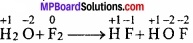Since, fluorine can undergo oxidation as well as reduction, it is an example of redox reaction.

Question 2.
In acidic medium MnO42- shows disproportionation reaction but MnO4 does not show why?
In oxidation state of Mn in MnO4-2 is +6. It can increase oxidation state (+7) and decrease (+4, +3, +2, 0 etc.). So in acidic medium it shows disproportionation reaction.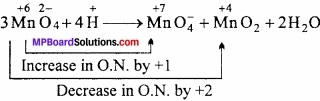In MnO4, Mn has maximum oxidation state (+7). It can only decrease its oxidation state. Due to which it cannot show disproportionation reaction.

Question 3.
In the following reaction whose oxidation and whose reduction occur:
PbS + 4H2O2 → PbSO4 + 4H2O
In this reaction PbS is oxidized as PbSO4 and H2O2 is reduced to form H2O.Question 4.
Differentiate between oxidation number and valency?
Differences between Oxidation number and Valency:
Oxidation:

1. It is the residual charge left on the atom of an element when all other atoms are removed from molecule or ion.
2. It refers to charge and can be positive, negative or zero, e.g., oxidation number of C in CO2 is +4 and that of oxygen is -2.
3. It may have fractional value.
4. Elements like C, N, O have variable oxidation number.

Valency:

1. It is the combining capacity of the element expressed as number of H – atom or double the number of oxygen atoms which combine with one atom of the element.
2. It refers to number only and does not carry any sign, e.g., in CO2 valency of carbon is 4 and that of oxygen is 2.
3. It is always a whole number.
4. Elements like C, N, O exhibit constant valency.

Question 5.
Why fluorine doesn’t show disproportionation reaction?
Like chlorine, bromine and iodine also undergo similar disproportionation reactions but fluorine does not. The reason for this anomalous behaviour is that fluorine being the strongest oxidizing agent does not show positive oxidation states. It, therefore, reacts in a different way forming oxygen difluoride (OF2).
2F2(g) + 2OH(g) → 2F(aq) + OF2(g) + H2O(l)

Question 6.
Show that galvanic cell where following reaction occurs:
Zn(s) + 2Ag+(aq) → Zn2+(aq) + 2 Ag(s)
Now explain :

1. Which electrode is -ve?
2. What is the motive of electricity in cell?
3. What is the reaction occurring on each electrode?

Galvanic cell Zn(s) ∥ Zn2+(aq)Ag(aq) ∥ Ag+(aq) ∥ Ag(s)

1. Zn electrode is negatively charged. Zn is oxidized into Zn+2.
2. Electricity flows from Ag electrode to Zn electrode and electrons flow from Zn to Ag electrode.
3. Reactions on electrode:

Cathode — Zn(s) → Zn+2(s) + 2e
Anode — 2Ag+(aq) + 2e → 2Ag(s)Question 7.
Write the definition of electrochemical cell? Write the chemical reactions of Daniel cell?
An electrochemical cell (Galvanic cell) is a device in which the redox reaction takes place indirectly and the decrease in free energy appears in the form of electrical work i.e. chemical energy is converted into electrical energy.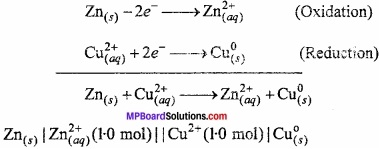Question 8.
Write the factors affecting electrode potential?
The factors affecting electrode potential are:

1. Tendency of metal to donate electrons: More the tendency to release electron more will be electrode potential.
2. Temperature: With increasing temperature electrode potential increases.
3. Concentration of solution: More the concentration of solution more will be electrode potential.

Question 9.
Determine the oxidation number of following:

1. Cr in K2Cr2O7
2. Mn in KMnO4
3. S in Na2S4O6.

Solution:
1. Cr in K2Cr2O7
2 (+1) + 2x + 7 (-2) = 0
or 2 + 2x – 14 = 0
or 2x = 12
or x = +6.

2. Mn in KMnO4
1 (+1) + 1x + 4(-2) = 0
or 1 + x – 8 = 0
or x = +7.

3. S in Na2S4O6
2 (+1) + 4x + 6 (-2) = 0
or 2 + 4x – 12 = 0
or 4x = 10
or x = 2.5.

Question 10.
Why does the following reactions occur:
XeO6(aq)4- + 2F(aq) + 6H+(aq) → XeO3(g) + F2(g) + 3H2O(l)?
What conclusion about the compound Na4XeO6 (of which XeO64- is a part) K2MnF6 can be drawn from the reaction?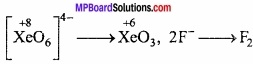O.N. of Xe decreases from +8 to +6. This shows that XeO64- is an oxidizing agent. It oxidises F to F2. This reaction shows that Na2XeO6 (or XeO6) is a stronger oxidizing agent than F2.

Question 11.
Chlorine is used to purify drinking water. Excess of chlorine is harmful. The excess chlorine is removed by treating with sulphur dioxide. Present a balanced equation for this redox change taking place in water?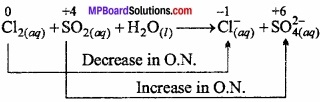Multiplying Cl by 2 (As 2 atoms are in Cl2)
Cl2(aq) + SO2(aq) + H2O(l) → 2Cl(aq) + SO42-(aq)
On adding 4H+ in left side and multiplying in H2O by 2,
Cl2(aq) + SO2(aq) + 2H2O(l) → 2Cl(aq) + SO42- + 4H+
It is a balanced disproportionation reaction.

Question 12.
Differentiate between electrochemical cell and electrolytic cell?
Differences between electrochemical cell and electrolytic cell:
Electrochemical (Galvanic) cell:

1. In Galvanic cell, chemical energy is changed into electrical energy.
2. Reaction in Galvanic cell is spontaneous.
3. Oxidation and reduction occurs in different half cell.
4. Anode is negative while cathode is positive terminal.
5. The electrons move from anode to cathode in the external circuit.

Electrolytic cell:

1. In electrolytic cell, electrical energy is changed into chemical energy.
2. Reaction in electrolytic cell is non – spontaneous.
3. Oxidation and reduction occurs in same container.
4. Anode is positive while cathode is negative terminal.
5. The electrons are supplied by the external circuit which enter through the cathode and came out through anode.Question 13.
What is Nernst equation? Derive relation between E and E°?
In Standard cell, the concentration of the solution used is 1M and the temperature is maintained at 25°C (For 298K). But the Galvanic cells are often constructed under non standard condition. In such cases the cell voltage (or EMF) is calculated by Nernst equation as follows:
E = E° – $$\frac{RT}{nF}$$ loge $$\frac { M_{ (s) } }{ M^{ n+ }_{ (aq) } }$$
or E = E° + $$\frac{RT}{nF}$$ loge $$\frac { M^{ n+ }_{ (aq) } }{ M_{ (s) } }$$
In changing loge in log10
or E = E° + $$\frac{2.303}{nF}$$ log10 $$\frac { M^{ n+ }_{ (aq) } }{ M_{ (s) } }$$ [M(s) = 1]
or E = E° + $$\frac{2.303RT}{nF}$$ log10[Mn+(aq)]
or E = E° + $$\frac{0.0591}{nF}$$ log10 [Mn+(aq)].

Question 14.
Consider the reactions:
2S2O32-(aq) + I2(s) → S4O62-(aq) + 2I(aq)
S2O32-(aq) → 2SO42-(aq) + 4Br(aq) + 10H+(aq)
Why does the same reductant, thiosulphate reacts differently with iodine and bromine?
Br2 is stronger oxidizing agent than I2, it oxidises SO32- to SO42- i. e. from +2 state to +6 state of sulphur. However, I2 being weaker oxidizing agent, oxidises S2O32- to S4O62- ion i.e. from + 2 to + 2.5 state of sulphur.

Redox Reactions Long Answer Type Questions – I

Question 1.
What is standard hydrogen electrode? How it is prepared?
Standard hydrogen electrode: This consists of gas at 1 atmospheric pressure bubbling over a platinum electrode immersed in 1 M HCl at 25°C (298 K) as shown in figure. The platinum electrode is coated with platinum black to atmospheric increase its surface. (MPBoardSolutions.com) The hydrogen electrode thus Pressure constructed forms a half cell which on coupling with any other half cell begins to work on the principle of oxidation or reduction. Electrode depending upon the 1 M H+solution circumstances works both as anode or cathode.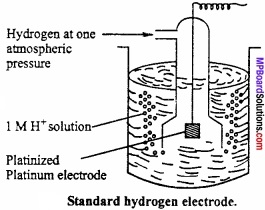Cell reaction of standard hydrogen electrode platinum electrode (SHE) when it acts as anode is
H2(g) → 2H+ + 2e
It is represented as
H2(g)(1 atm) Pt | H3O+(aq)(1.0 M)
When it acts as cathode, the cell reaction is
2H+ + 2e → H2(aq)
and it is represented as
H3O+(aq) (1.0 M) | H2(g) (1 atm) Pt
Standard hydrogen electrode (SHE) is arbitarily assigned a potential of zero.

Question 2.
On the basis of electrode potential explain which reaction is feasible:
Cu2+/Cu = 0.34 V, E°Zn2+/Zn = -0.76 V, E°Mg2+/Mg = 2.37 V, E°Fe2+/Fe = -0.74V

1. Cu + Zn2+ → Cu2+ + Zn
2. Mg + Fe2+ → Mg2+ + Fe.

Solution:
1. Cu + Zn2+ → Cu2+ + Zn
Cu2+/Cu = 0.34 V and E°Zn2+/Zn = – 0.76 V
In given cell, Cu oxidised to Cu2+. So Cu2+/Cu will act as anode and Zn2+/Zn acts as cathode.
cell = E°cathode – E°anode
cell = – 0.74 – (-2.37) = +1.63V
cell = +Ve, shows that the reaction is feasible.

2. Mg + Fe2+ → Mg2+ + Fe
Mg2+/Mg = 2.37 V and E°Cu2+/Cu = – 0.74 V
Mg oxidised to Mg2+ and acts as anode Mg2+/Mg.
Fe2+ reduced to Fe and acts as cathode Fe2+/Fe.
cell = E°cathode – E°anode
cell = – 0.74 – (-2.37) = +1.63 VQuestion 3.
While sulphur dioxide and hydrogen peroxide can act as oxidizing as well as reducing agents in their reactions, ozone and nitric acid act only as oxidants, why?
In sulphur dioxide (SO2) and hydrogen peroxide (H2O2), the oxidation states of sulphur and oxygen are +4 and -1 respectively. Since, they can increase as well as decrease when there compounds take part in chemical reaction, they can act as oxidizing as well as reducing agents. For example,
Increase in O.N.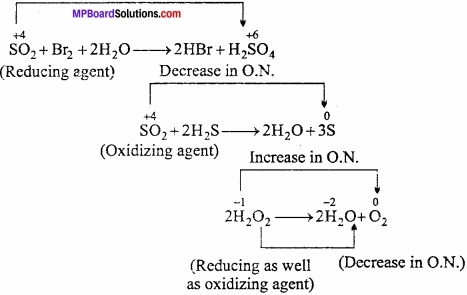In ozone (O3), the oxidation state of oxygen is zero while in nitric acid (HNO3), the oxidation state of nitrogen is +5. Since, both of them can undergo decrease in oxidation state and not an increase in its value, they can act only as oxidizing agents and not as reducing agents.

In ozone (O3), the oxidation state of oxygen is zero while in nitric acid (HNO3), the oxidation state of nitrogen is + 5. Since both them can undergo decrease in oxidation state and not an increase in its value, they can act only as oxidizing agents and not as reducing agents.Question 4.
How do you count for the following observation though alkaline potassium permanganate and acidic potassium permanganate both are used as oxidants, yet in manufacture of benzoic acid from toluene we use alcoholic potassium permanganate as an oxidant, why? Write a balanced redox equation for the reaction?
Toluene can be oxidized to benzoic acid in acidic, alkaline and neutral medium by using potassium permanganate.

1. In acidic medium:
[MnO4(aq) + 8H+(aq) + 5e → Mn2+(aq) + 4H2O(l)] × 6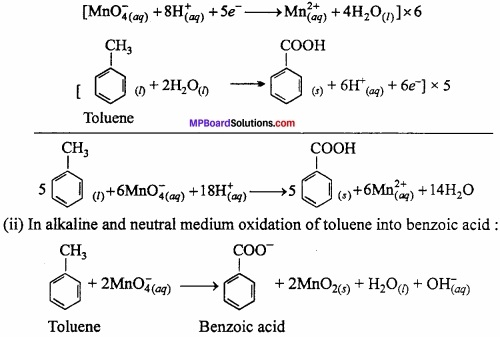In the manufacture of benzoic acid alcoholic KMnO4 is more useful.
Alcohol if used as solvent will help in the formation of a homogeneous mixture between toluene (non – polar) and KMnO4 (ionic). Actually alcohol has non – polar alkyl group as well as polar OH group.

Question 5.
How the redox reactions are balanced by ion – electron method?
The redox reactions are balanced by ion – electron method are as follows:
1. Indicate oxidation number of each element and identify the elements which undergoes change in oxidation number.

2. Indicate increase and decrease in oxidation number per atom. Multiply the increase or decrease in oxidation number with number of atoms undergoing the change.

3. Multiply the formula with suitable integer to equalise the increase and decrease in oxidation number.

4. Balance O atoms:
K2Cr2O7 + 6HCl + xHCl → 2KCl + 2CrCl3 + yCl2 + H2O + 6H2O

5. Balance H atoms:
K2Cr2O7 + 6HCl + 8 HCl → 2KCl + 2CrCl3 + 3Cl2 + 7H2O
Here x = 8 and y = 3 to balance Cl atoms
K2Cr22-O7 + 14 HCl → 2KCl + 2rCl3 + 3Cl2 + 7H2O.
Example 2. Cr2O72- + Fe2+ + H+ → Cr3+ + Fe3+ + H2O.
Solution:
Step 1. (Cr2O7)2- + Fe2+ + H+ → Cr3+ + Fe3+ + H2O.

Step 2.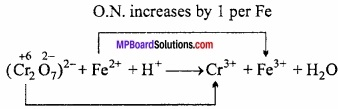Step 3.
Multiply Fe by 6 to equalise increase or decrease in O.N.
Cr2O72- + 6Fe2+ + H+ → Cr3+ + Fe3+ + H2O

Step 4.
Balance atoms other than O and H
Cr2O72- + 6Fe2+ + H+ → 2Cr3+ + 6Fe3+ + H2O

Step 5.
Balance O atoms
Cr2O72- + 6Fe2+ + H+ → 2Cr3+ + 6Fe3+ + H2O + 6H2O

Step 6.
Balance H atoms
Cr2O72- + 6Fe2+ + 14H+ → 2Cr3+ + 6Fe3+ + 7H2O.

Question 6.
On the basis of electrode potential values given below? Determine reaction between reactants will occur or not?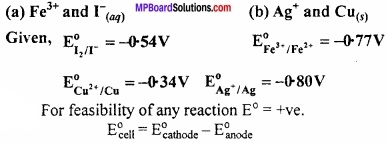For feasibility of any reaction E° = +ve.
cell = E°cathode – E°anode
(a) Fe3+(aq) and I(aq):
2Fe3+ + 2I(aq) → 2Fe2+(aq) + 2I2g
Half cell reaction (oxidation)
2I(aq) → I2(g) + 2e E° = – 0.54 V
Reducion half cell,
2Fe3+(aq) + 2e → 2Fe2+(aq) E° = +0.77 V
Complete reaction,
2Fe3+(aq) + 2I(aq) → 2Fe2+(aq) + I2(g)cell = +0.23 V
E° = +ve, reaction is feasible.

(b) Ag+(aq) and Cu(s)2+:
2Ag(aq) + Cu(s) → Cu2+(aq) + 2Ag(aq)
Oxidation half reaction,
Cu(s) → Cu2+(aq) + 2e E° = – 0.34 V
Reduction half reaction,
2Ag+(aq) + 2e → 2Ag(s) E° = – 0.80 V
Complete reaction,
Cu(s) + 2Ag+(aq) → Cu2+(aq) + 2Ag(s)cell = +0.46 VQuestion 7.
Give the main characteristics of electrochemical series?
The main characteristics of electrochemical series are:

1. Tendency to loose electron becomes greater and the corresponding element or ion behaves as strong reducing agent if it has greater negative value of E°.
2. The substances above hydrogen are weak oxidising agents and substances below it are strong oxidising agents.
3. If the reducing agent of electrode pair is strong reducing agent, its oxidising agent is weak and when reducing agent of electrode pair is weak, its oxidising agent is strong.
4. In series the reducing efficiency decreases from above to downwards.
5. The more the positive value of E°(MPBoardSolutions.com) the stronger is its oxidising properties. In the series the oxidising power of cation decreases as we move upwards from downwards.
6. In series the electropositive character of metals decreases from upwards to downwards.
7. Along the series the electronegative character of non – metal increases from upward to downwards.
8. The metals which come prior to hydrogen displaces hydrogen from acids,
9. The metals which occupy higher position in the series displaces metals which come later in series from their salt solution.

Question 8.
Balance the equation by oxidation number method and identify the oxidising agent and reducing agent?
Cl2O7(g) → ClO2(aq) + O2(g).
Oxidation number method: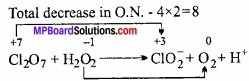Balance the increase and decrease in O.N. multiply H2O2 by 4,
Cl2O7 + 4H2O2 → ClO2 + O2
Balance atom other,
Cl2O7(g) + 4H2O2(aq) → 2ClO2(aq) + 4O2(g)
Balance ‘H’,
Cl2O7(g) + 4H2O2(aq) + 2OH(aq) → 2ClO2(aq) + 4O2(g) + 5H2O.

Question 9.
Whenever a reaction between an oxidizing agent and a reducing agent is carried out compound of lower oxidation state is formed if the reducing agent is ¡n excess and compound of higher oxidation state is formed if the oxidising agent is in excess. Justify this statement by giving three illustrations?
1. Let us consider the reaction between carbon and oxygen.
Reducing agent + Oxidising agent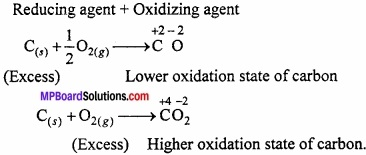2. Let us consider the reaction between white phosphorous (P4) and Cl2(g)3. Let us consider the reaction between sulphur and oxygen.
Reducing agent + Oxidising agent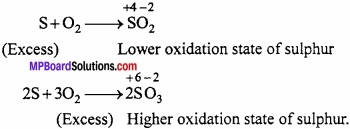Redox Reactions Long Answer Type Questions – II

Question 1.
Balance the following reaction by ion – electron method:
Cr2O72- + Fe2+ + H+ → Cr+3 + Fe+3.
Solution:
Cr2O72- + Fe2+ → Cr+3 + Fe+3 + H2O

1. Write the oxidation number of each atom in skeleton equation.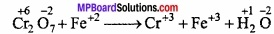Species undergo change is Cr and Fe.

2. Dividing the equation into two half reaction.
Cr2O72- → Cr+3 (Reduction half)
Fe+2 → Fe+3 (Oxidation half)

3. Balancing reduction half:
(a) Balancing Cr atom,
Cr2O72- → 2Cr+3

(b) Adding e to make up the difference in O.N.(Since each Cr atom gain 3e ∴ for 2 Cr atom 6e)

(iv) Balancing oxidation half:
(a) Balancing Fe atom (Balanced)
(b) Adding e to make up the difference in O.N.
Fe+2 → Fe+3 + e

(v) Balancing ‘O’ atom,
Cr2O72- + 6e → 2Cr+3 + 7H2O
Fe+2 → Fe+3 + e

(vi) Balancing H by adding H+ ions to the side which is deficient on H atoms.
Cr2O72- + 6e + 14H+ → 2Cr+3 + 7H2O
Fe2+ → Fe+3 + e

(vii) Equalising e,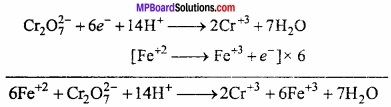Question 2.
To determine the oxidising power/reducing power in a solution which method is used? Explain with example?
Determination of standard electrode potential ; To determine electrode potential of an electrode, a cell is setup using this electrode and standard hydrogen electrode. The EMF of the cell is measured with the help of a voltameter (or more accurately by potentiometer). (MPBoardSolutions.com) The electrode potential of hydrogen electrode is taken as zero. Therefore, the E.M.F. of such a cell will directly give the value for electrode potential for the given electrode at standard condition (298 K, 1M, 1 atm pressure).

1. If the deflection in galvanometer is towards metal electrode (experimental electrode) then it is anode (-ve terminal) of the cell. It is allotted negative value of standard reduction electrode potential.
For example, in the determination of E° value of zinc, zinc acts as anode and has got negative value of E°.cell = E°cathode – E°anode
∴ 0.76 V = 0.00V – E°zn+2/Zn = -0.76V

Question 3.
What is oxidation number? Write its main rules of determination?
Oxidation number of an element is defined as, “The residual charge left on its atom when all the other atoms are removed from the molecule as ions”. Atoms can have positive, negative or zero oxidation state depending upon their state of combination. (MPBoardSolutions.com) In fact, oxidation number is the charge assigned to the atom in a species according to some rules. It is also known as oxidation state.

Rules for assigning oxidation number:
The assignment of oxidation numbers is arbitrary and is usually governed by the following conclusions:

1. The oxidation number of an element in free state i. e., elementary state is regarded as zero. For example,
Oxidation state of hydrogen in H2 = 0
Oxidation state of helium in He = 0
Oxidation state of sulphur in S8 = 0
Oxidation state of sodium in Na = 0
Oxidation state of magnesium in Mg = 0

2. In a compound, the more electronegative elements are assigned negative (-) oxidation state and less electronegative elements are assigned positive (+) values. For example, in HCl as chlorine is more electronegative than hydrogen its oxidation state will have negative value while that of hydrogen will be positive.

3. In the formula of a compound, the sum of the negative oxidation states is equal to the sum of the positive oxidation states.

4. The sum of oxidation number of all the atoms in a neutral molecule is taken as zero.

5. Hydrogen has an oxidation state +1 in compounds like H2S, H2O, HCl, etc. Exceptionally it has the oxidation number -1 in metallic hydrides, such as NaH, CaH2, etc.

6. Oxygen is usually assigned oxidation number -2, except in H2O2 and in oxide of fluorine [F2O], in which the oxidation number -1 and +2 respectively. In all, oxidation number of oxygen is -1.

7. Fluorine being the most electronegative element is assigned oxidation number -1 in all its compounds. Other halogens also show -1 oxidation number.

8. The number of monoatomic ion in an ionic compound is equal to its electric charge. Thus, the elements of group IA of the periodic table (Li, Na, K, Rb, Cs) all have oxidation number + 1, while the alkaline earth metals of group IIA (Ca, Sr, Ba) have oxidation number + 2.Question 4.
Balance the following reaction by ion – electron method?
Ion – electron method:
Oxidation half equation is: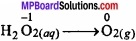The oxidation number is balanced by adding 2 electrons as:
H2O2(aq) → O2(g) + 2e
The charge is balanced by adding 2OH ions as:
H2O2(aq) + 2OH(aq) → O2(g) + 2e
The oxygen atoms are balanced by adding 2H2O as:
H2O2(aq) + 20H(aq) → O2(g) + 2H2O(s) + 2e ……………… (1)
The reduction half equation is:The Cl atoms are balanced as:
Cl2O7(g) → 2ClO2(aq)
The oxidation number is balanced by adding 8 electrons as:
Cl2O7(g) + 8e → 2ClO2(aq)
The charge is balanced by adding 6OH as:
Cl2O7(g) + 8e → 2ClO2(aq) + 6OH(aq) ………………………… (2)
The balancing equation can be obtained by multiplying equation (1) with 4 and adding equation (2) to it as:
Cl2O7(g) + 4H2O2(aq) + 2OH(aq) → 2ClO2(aq) + 4O2(g) + 5H2O(l)

Question 5.
Write the main uses of electrochemical series?
Electrochemical series:
When different elements and ions are arranged in increasing order of their standard electrode potentials, a series is obtained which is called electrochemical series or activity series.
The uses of electrochemical series are:
1. Electropositive character of metal:
Metal which loses electron easily from its outermost shell shows high electropositive character while the atom which loses electron with difficulty shows least electropositive character. In electrochemical series, Li shows maximum electropositive character while fluorine shows least electropositive character.

2. Comparison of reactivity of metals:
Metal having negative value of E° loses electron easily. Hence, greater the negative value of E° more is the reactivity and reducing character of a metal.

3. Knowledge of oxidizing agent and reducing agent:
Substance which gains electron and shows maximum value of standard electrode potential E° is strongest oxidizing agent. On the basis of this F is strongest oxidizing agent while Li is weakest oxidizing agent.

4. Displacement of elements:
Metal having greater tendency to form ion displaces others. Therefore, elements getting priority in the series displace ions of the elements following them. For example. When Cu turnings are added to AgNO3 solution, Ag gets precipitated.
2AgNO3(aq) + Cu(s) → Cu(NO3)2(aq) + 2Ag(s)

5. Electroplating:
Process of depositing layer of gold or silver on copper, brass, iron etc. is called electroplating. It makes articles lustrous and attractive.

6. Metallurgy:
More reactive metal displaces less reactive metal from their aqueous salt solution.

7. Corrosion of metals:
Destruction of metals due to action of chemicals, air and moisture is called corrosion.
e.g., iron rust in moist air. To prevent this a layer of more reactive metal like Sn or Zn is deposited on iron. Coating iron with zinc is called galvanization.Question 6.
What information did you get from the following reaction:
(CN)2(g) + 20H(aq) → CN(aq) + CNO(aq) + H2O(l)
(CN)2(g) + 2OH(aq) → CNO(aq) + H2O(l).
1. Let the O.N. of C in (CN)2 = x
2x + 2 (-3) = 0
or x = +3.

2. Let the O.N. of C in CN = x
x + (-3) = -1
or x = +2.

3. Let the O.N. of C in CNO = x
x + (-3) + (-2) = -1 or
x = +4.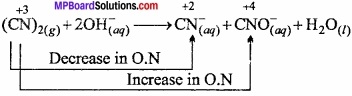Following informations obtained from above equation:

1. It is a disproportionation reaction.
2. The reaction occurs in basic medium.
3. O.N. of N in (CN)2 is -3 and that in CN is -2 and in CNO it is -5.
4. Cyanogen (CN)2 gets simultaneously reduced to CN ion as well as oxidized to cyanate, CNO ion.

Question 7.
The Mn3+ ion is unstable in solution and undergoes disproportionation to give Mn2+, MnO2 and H+ ion. Write a balanced ionic equation for the reaction?